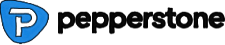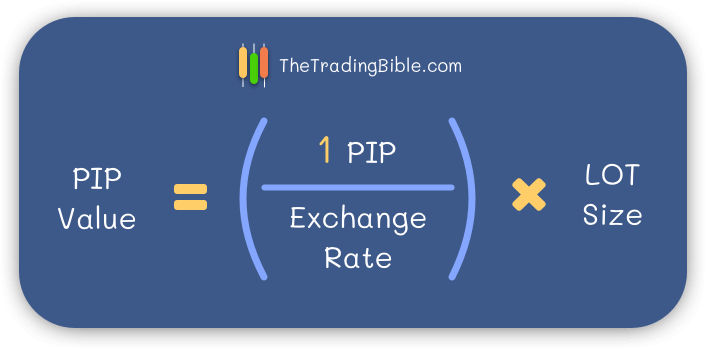Compare Brokers

Vs.

# How Much is 0.01 Lot Size in Forex Trading?

By Stefano Treviso , Updated on: Apr 07 2023.

0.01 Lot Size in Forex trading (also known as Micro Lot) equals 1.000 units of any given currency. In any forex pair where the quote currency is the USD such as the GBP/USD, the pip value per Micro Lot is \$0.1.

If you’re trading a Micro lot of GBP/USD and the price moves 50 Pips in your favour then to calculate your profit, multiply 50 pips times \$0.1, the result is a profit of \$5.

Understanding Lot Sizes is the key to efficient trading as you'll be able to determine your risk-reward ratios for an effective forex risk management strategy and your costs as well. A good example of this would be:

• Suppose that there is a currency pair that only moves 1 Pip a week
• If in this imaginary trade, your Pip Value per Lot size is \$5 and the spread your broker charges is 5 Pips, there is no way to be profitable
• Every time you open a 1 Lot trade, you're paying \$50 in commissions and based on this imaginary example, your best odds (due to the slow currency pair price variation) is making \$5 a week

See how it makes sense? Well, time to continue reading so you can learn how to improve your forex trading and which low spreads forex brokers can greatly improve your cost efficiency.

## Featured Low Spreads Forex Brokers74% of retail CFD accounts lose money.

Dynamic - From 1 Pip74-89% of retail CFD accounts lose money.

Dynamic - From 0 to 0.77 Pips

## Forex Lot Sizes Basics

A standard lot in Forex trading equals 100.000 units of any given currency, to arrive at the calculation of how much is 0.01 Lot all we need to do is multiply 0.01 times 100.000, the result is 1.000.

The most common types of Lot sizes in Forex are:

As we understood a Mini lot is worth 1.000 units of any given currency which can also be referred to as 0.01 lot in forex. So if we buy 0.01 lots of EUR/USD that means that we purchased €1.000 worth of USD.

## Pip Value per Lot Size

So far we described the pip value per micro lot (0.01 lot) using forex pairs where the quote (secondary currency) is the USD.

In case you’re wondering how to calculate the pip value per lot size when there are different currency combinations where the USD is not the quote, you can use the following formula:Explaining the formula:

• 1 PIP: in here you insert the value of the pip for the currency pair you’re trading, in most cases it’s 0.0001, in the case of the USD/JPY is 0.01)
• Exchange Rate: current price of your currency pair
• Lot size: the actual number of currency units depending on your lot size, for example: 100.000, 10.000, 1.000, 100

The results of the Pip value per Lot size formula always come expressed in terms of the base currency which then you can convert into the currency of your choice (usually everyone converts to USD), the only moment where you don’t need to do this conversion is if the pair has the USD as base (first currency) , as the result of the formula comes expressed in terms of the base.

Remember, when the currency pair has the USD as secondary currency, you don’t need to do the formula, you already know that:

• PIP Value per Standard lot on pairs with USD as quote: \$10
• PIP Value per Mini lot on pairs with USD as quote: \$1
• PIP Value per Micro lot on pairs with USD as quote: \$0.1
• PIP Value per Nano lot on pairs with USD as quote: \$0.01

For all others, use the formula and you’ll be able to calculate your pip value per lot size regardless of the currency pair you’re trading.